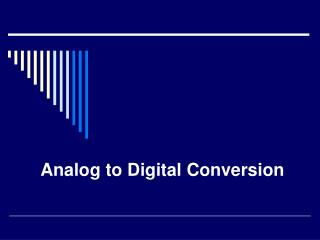DownloadDownload PresentationAnalog to Digital Conversion

# Analog to Digital Conversion

Download Presentation## Analog to Digital Conversion

- - - - - - - - - - - - - - - - - - - - - - - - - - - E N D - - - - - - - - - - - - - - - - - - - - - - - - - - -
##### Presentation Transcript

1. Analog to Digital Conversion

2. Introduction • An analog-to-digital converter(ADC, A/D, or A to D) is a device that converts continuous signals to discrete digital numbers • In electronics, a digital-to-analog converter(DAC or D-to-A) is a device for converting a digital (usually binary) code to an analog signal (current, voltage or charges). Digital-to-Analog Converters are the interface between the abstract digital world and the analog real life. Simple switches, a network of resistors, current sources or capacitors may implement this conversion

3. Important terminologies in ADC • Resolution • Response type • Linear ADCs • Non-linear ADCs • Accuracy • Sampling rate • Aliasing

4. Resolution • The resolution of the converter indicates the smallest analog value that it can convert to a digital number • If the ADC has 8 bits and the Full scale is 0-5 Volts, then the ADC voltage resolution is: • 5/28 = 0.01953125 Volts

5. Response type • Linear ADCs • Output binary value changes approximately with the analog value within the resolution (or ½ the resolution) • Non-linear ADCs • Uses techniques known as companding to ‘magnify” the low amplitude analog signals • m-law • A-law • Dolby

6. Accuracy • Accuracy depends on • Quantization error • Non-linear error caused by the physical imperfections of ADC

7. Sampling rate • For ADC, a signal values are measured and stored at intervals of time Ts, the sampling time. • A bandlimited analog signal must be sampled at a frequency fs = 1/Ts that is twice the maximum frequency (fa) of the bandlimited signal • fs = 2fa is known as the Nyquist Sampling frequency

8. Aliasing • If a signal values are measured and stored at frequencies greater than the Nyquist sampling rate, the signal can be reproduced exactly (within quantization and other non-linear error accuracy). • However, If a function is sampled at less than Nyquist rate, the resulting function may have different frequency content. This is known as aliasing. • For example: If a 3 KHz sine wave is sampled at 4 KHz, the resulting signal will appear as a 1 KHz signal.

12. Analog to Digital chip: ADC0820 • 8-Bit High Speed µP Compatible A/D Converter with Track/Hold Function • Uses ½ flash conversion technique • consists of 32 comparators • a most significant 4-bit ADC • a least significant 4-bit ADC • 1.5 µs conversion time • Does not need external sample-and-hold for signals moving at less than 100 mV/µs.

14. Analog to Digital chip: ADC0820 • Has many input modes, RD, WR-RD, WR-RD Standalone • Input pulse required to read analog data (Sample) • Must sample at more than Nyquist rate (fs = 2*fa) • Outputs signal when data is valid